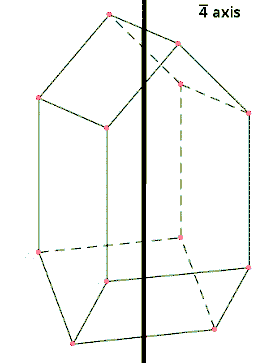# Packing in solids

Packing of spheres can describe the solid structures of crystals. In a crystal structure, the centers of atoms, ions, or molecules lie on the lattice points.

Atoms are assumed to be spherical to explain the bonding and structures of metallic crystals. These spherical particles can be packed into different arrangements. In closest packed structures, the arrangement of the spheres are densely packed in order to take up the greatest amount of space possible.

## Types

### Close Packed Structure

Matters exist in solid state because of close packing of their constituent particles. There are two types of close packing found in solids. These are Cubic Close Packed (ccp) and Hexagonal Close Packed (hcp) lattice.#### Cubic Close packed (ccp):

In this type of packing, the spheres of molecules are adjacent to each other that each row of spheres in a particular dimension is a repetition of the pervious row. The spheres of a particular row don’t fit in the depressions between two adjacent spheres of the previous row.

#### Hexagonal Close packed (hcp):

In this type of packing, the spheres of molecules of a particular row in a particular dimension are in a position that they fit into depressions between adjacent spheres of the previous row. This type of arrangement is called ABAB type arrangement. This type of packed lattice is found in many metals such as magnesium, zinc, etc.

#### Coordination number:

The number of adjacent particles of atoms is called coordination number.

Example –

If cation of an ionic solid occupies all the octahedral voids, then the formula of the compound can be obtained as follows: Let ‘A’ are cations and ‘B’ are anions in the compound.

Since the number of close packed sphere is equal to the number of octahedral voids formed, thus the cations and anions must be in the ratio of 1:1.

Therefore, A and B will be combined in the ratio of A:B.

Thus the formula of the compound will be AB.